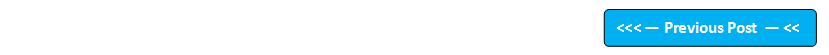## Blog Pages

### 44. Assigning values to variables of different data typeThe following are the example programs for demonstrating what happens when the variable's data type and the assigning value's data type don't match (Some cases throws errors where as some cases will convert the values).

 Program to demonstrate what happens on providing a decimal value to a variable which is declared as an int data type. class IntVariableDecimalValue {    public static void main(String args[])    {         int a=123.456;   // We have assigned a decimal value to a variable which is declared as int         System.out.println(a);    }  } Output of this program: Error displayed while compiling the program. "Required int but found double" This means that Java is telling us to provide the integer value instead of double (i.e. decimal) value. Program to demonstrate what happens on providing an integer value to a variable which is declared as an double data type. class DoubleVariableIntegerValue {    public static void main(String args[])    {          double a=123;    // We have assigned an integer value to a variable which is declared as double          System.out.println(a);    }  } Output of this program: 123.0 This means that Java will automatically assign 123.0 instead of 123 to the variable which is declared as double Program to demonstrate what happens on providing a character value to a variable which is declared as int data type class IntVariableCharValue {    public static void main(String args[])    {           int a='x';   // We have assigned a character value to a variable which is declared as int           System.out.println(a);    }  } Output of this program: 120 This means that Java will automatically convert the char value to Unicode value and assign it to the variable which is declared as int Program to demonstrate what happens on providing a character value to a variable which is declared as boolean data type class BooleanVariableCharValue {      public static void main(String args[])     {          boolean a='x';   // We have assigned a character value to a variable which is declared as boolean          System.out.println(a);    }  } Output of this program: Error displayed while compiling the program. "Required boolean but found char" This means that Java is telling us to provide the boolean value instead of char value. Program to demonstrate what happens on providing a long integer value to a variable which is declared as short data type class ShortVariableIntValue {      public static void main(String args[])     {          short a=1234567;   //Assigning an int value to a variable which is declared as short          System.out.println(a);      }  } Output of this program: Error displayed while compiling the program. "Required short but found int" This means that Java is telling us to provide the short value instead of int value. Program to demonstrate what happens on providing an integer value to a variable which is declared as byte data type class ByteVariableIntValue {     public static void main(String args[])    {         byte a=12345;   //Assigning an int value to a variable which is declared as byte         System.out.println(a);    }  } Output of this program: Error displayed while compiling the program. "Required byte but found int" This means that Java is telling us to provide the byte value instead of int value. Program to demonstrate what happens on providing an integer value of size more than 10 digits to a variable which is declared as long data type class LongVariableLongValue {    public static void main(String args[])    {          long a=1234567891112;  // Assigning a 13 digit integer value to a long declared variable          System.out.println(a);    }  } Output of this program: Error displayed while compiling the program. "integer number too large" This means in Java you cant assign an integer value of more than 10 digit size to a long declared variable. But the variable declared as long can hold more than 10 digit number if the value comes from an operation done as shown in the next example. Program to demonstrate what happens when a variable declared as long gets an integer value of more than 10 digit in size from an operation class LongVariableLongValueFromOperation {    public static void main(String args[])    {          long a=123456789, b=123456789, c;   // Assigning  integer values of 10 digit size to a and b variables          c=a*b;  // c is a variable which is declared as long in the earlier step and will be assigned an integer value which is a result of  a*b (The result of this operation is an integer number of size more than 10 digits)          System.out.println(c);    }  } Output of this program: 15241578750190521   This means the variable c which is declared as long is now holding an integer number which is of 17 digit size. Program to demonstrate what happens when a variable declared as char is assigned a boolean value class CharVariableBooleanValue {    public static void main(String args[])    {         char a=true;    // Assigning a boolean value true to the variable which is declared as char         System.out.println(a);    }  } Output of this program: Error displayed while compiling the program. "Required char but found boolean" This means that Java is telling us to provide the char value instead of boolean value.

Variable Declaration and Initialization topic will be explained in the next post.